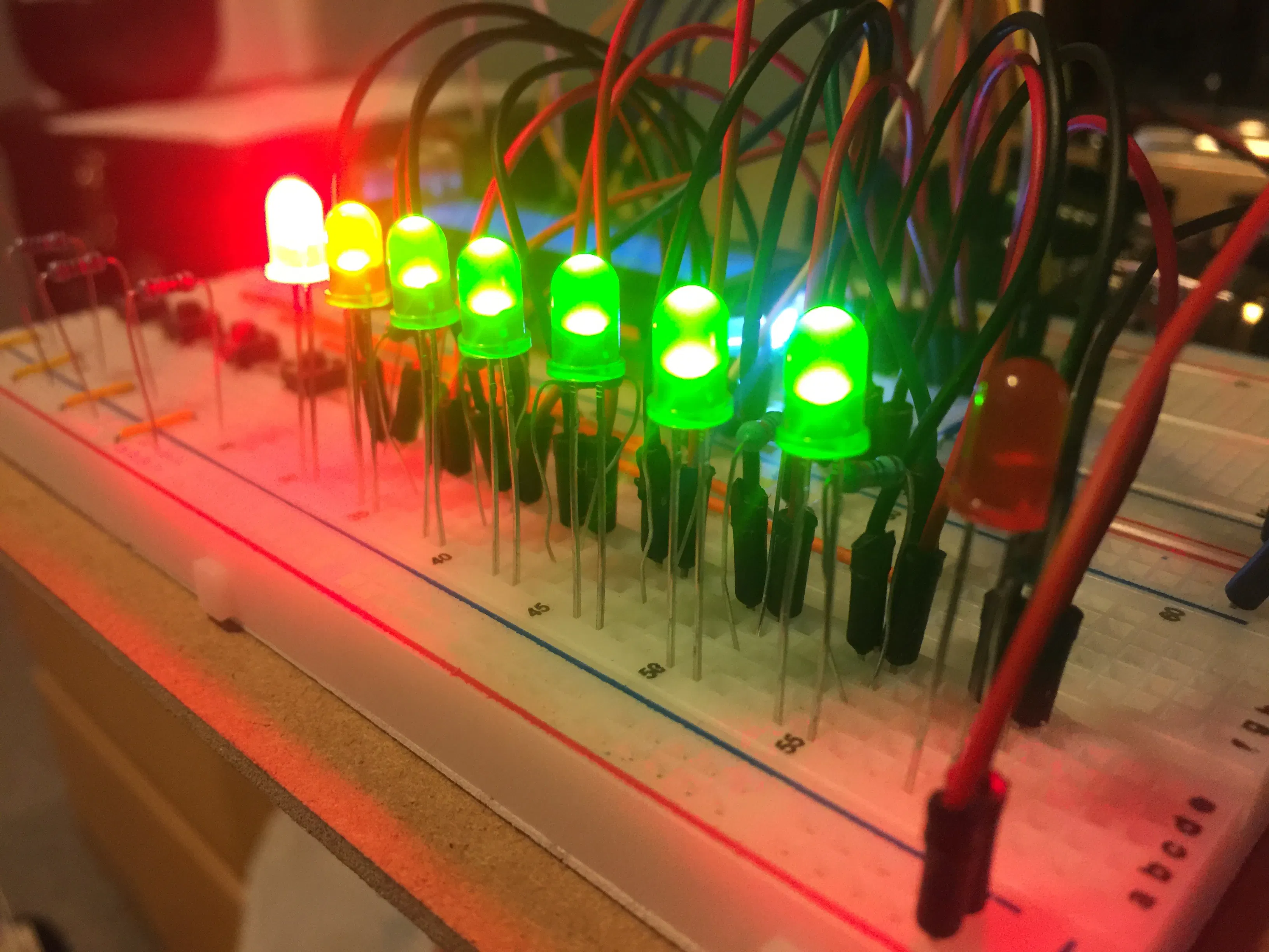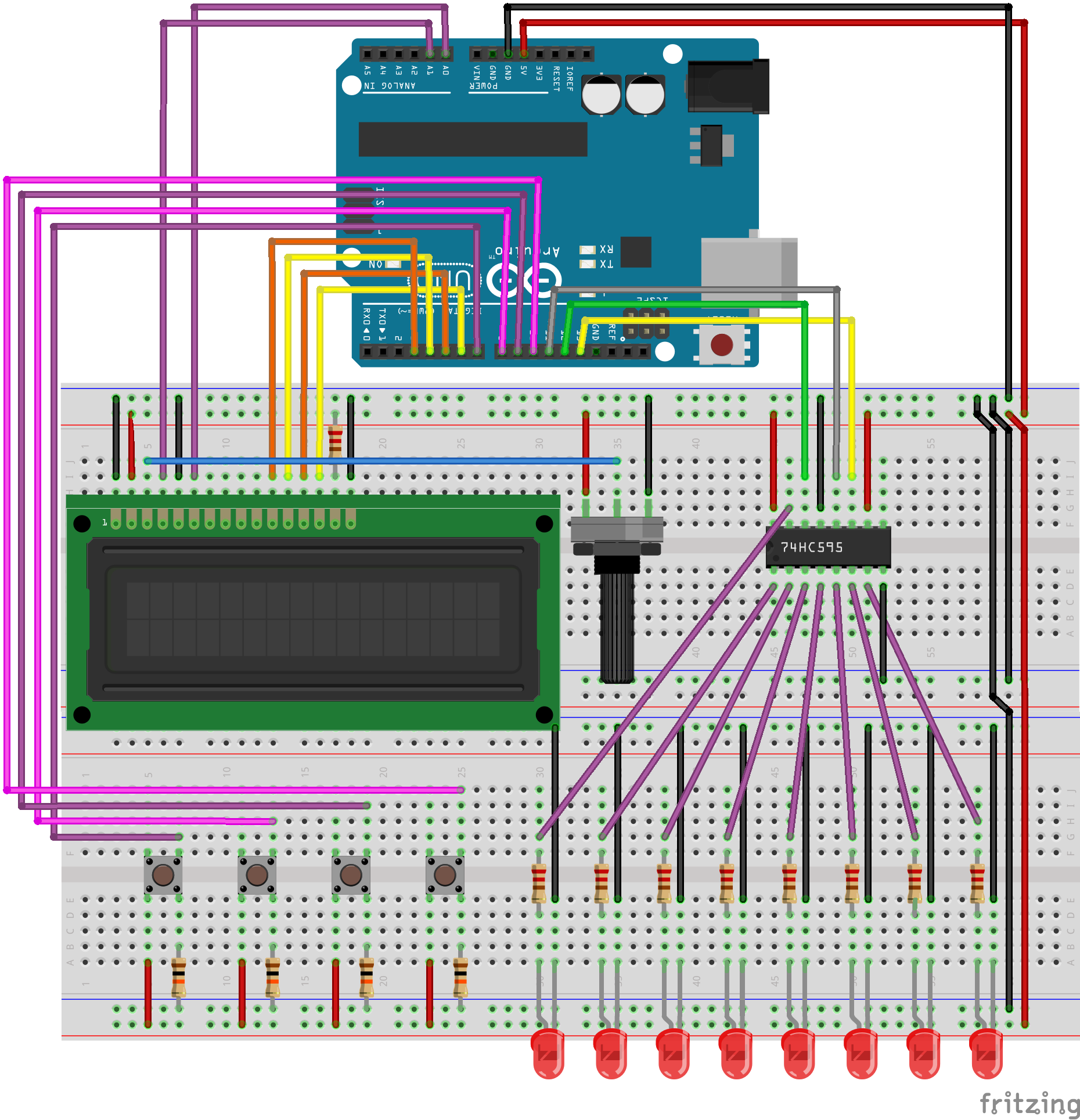Project tutorial# Light Sequence Creator © GPL3+

This project allows the user to create their own light animations! Can modify each frame, the speed, and loop count.

• 21,467 views
• 43 respects

 hands

## Apps and online services

I have been having fun with lights and arrays and thought I would make use of the shift register and the LCD screen to create a program in which you can modify and display your light animations without having to go into the code and create an animation by hand each time.

There are 4 buttons, from left to right they are numbered 1 - 4. They allow you to navigate the menu, and change the variables. Button 1 is used for incrementing values and for turning the state of a light to HIGH. Button 2 is used for decrementing values, and for turning the state of a light to LOW. Button 3 can move the cursor backwards while making a frame, and button 4 is used as a 'back' button.

The program can modify the speed of the animation and the amount of times it will loop. I might release another version allowing multiple animations.

You can change frames by changing the ARR_SIZE at the top in the code

Enjoy

## Code

##### Light SequencerC/C++
Plug this code in and run it, if the wiring is correct then it should NOT have issues!
Enjoy
```#include <LiquidCrystal.h>

#define ARR_SIZE 16 // CHANGE this to any number of frames
#define LIGHTS 8

// Controls the game button inputs
const int btn1 = 7, btn2 = 8, btn3 = 9, btn4 = 10;
// Variable for reading the pushbutton status
int bs1 = 0, bs2 = 0, bs3 = 0, bs4 = 0;

// Pin connected to ST_CP of 74HC595
const int latchPin = 11;
// Pin connected to SH_CP of 74HC595
const int clockPin = 13;
// Pin connected to DS of 74HC595
const int dataPin = 12;

// Holders for information you're going to pass
byte data;
// Used to read either 1 or 0
bool ArrOne[ARR_SIZE][LIGHTS] = {false}; // animation
// Translated for the register as bytes
byte lightArrOne[ARR_SIZE] = {0xFF};

// Variables to affect the animation
int animSpeed = 50; // ms between each frame
int loopCount = 5; // amount of times it will run

// Controls LCD Values
const int rs = 15, en = 14, d4 = 3, d5 = 4, d6 = 5, d7 = 6;
LiquidCrystal lcd(rs, en, d4, d5, d6, d7);

void setup()
{
// Set each button up
pinMode(btn1, INPUT_PULLUP);
pinMode(btn2, INPUT_PULLUP);
pinMode(btn3, INPUT_PULLUP);
pinMode(btn4, INPUT_PULLUP);

// Set up the LCD's number of columns and rows:
lcd.begin(16, 2);

// Set register latch
pinMode(latchPin, OUTPUT);

}

void loop()
{

// Play the anim
if(bs1 == HIGH)
{
bs1 = LOW;// just used to prevent bad/double input
delay(150);
Play();

// Modify the anim
} else if(bs2 == HIGH)
{
bs2 = LOW;
delay(150);
Create(ArrOne);

// Change the speed of the anim
} else if(bs3 == HIGH)
{
bs3 = LOW;
delay(150);
SetSpeed();

// Change the number of times the anim will play
} else if(bs4 == HIGH)
{
bs4 = LOW;
delay(150);
SetLoop();
}
delay(150);
}

// Function to print info for the main menu
{
ClearScreen();
lcd.print("1-Play   3-Speed");
lcd.setCursor(0, 1);
lcd.print("2-Create 4-Loops");
}

// Function to print info for creating a frame
void PrintCreate(bool Arr[][LIGHTS], int frame, int _bit)
{
ClearScreen();
lcd.print("Frame:");
lcd.print(frame+1);
if(frame < 9)
lcd.print(" ");

for(int j = 0; j < _bit; j++)
lcd.print(" ");
lcd.print("v");

lcd.setCursor(0, 1);
lcd.print("        ");
for(int j = 0; j < 8; j++)
lcd.print(Arr[frame][j]);
}

// Function to print info for setting the speed
void PrintSetSpeed()
{
ClearScreen();
lcd.print("Set Speed(ms)");
lcd.setCursor(0, 1);
lcd.print(animSpeed);
}

// Function to print info for changing the number of animation loops
void PrintSetLoop()
{
ClearScreen();
lcd.print("Set Loop Count");
lcd.setCursor(0, 1);
lcd.print(loopCount);
}

// Function to play the animation with the given parameters
void Play()
{
// The animation
for(int count = 0; count < loopCount; count++)
{
for(int i = 0; i < ARR_SIZE; ++i)
{
//load the light sequence you want from array
data = lightArrOne[i];
//ground latchPin and hold low for as long as you are transmitting
digitalWrite(latchPin, 0);
//move 'em out
shiftOut(dataPin, clockPin, data);
//return the latch pin high to signal chip that it
//no longer needs to listen for information
digitalWrite(latchPin, 1);

delay(animSpeed);
}
}
data = 0;
for(int i = 0; i < ARR_SIZE; ++i)
{
//ground latchPin and hold low for as long as you are transmitting
digitalWrite(latchPin, 0);
//move 'em out
shiftOut(dataPin, clockPin, data);
//return the latch pin high to signal chip that it
//no longer needs to listen for information
digitalWrite(latchPin, 1);
}
}

// Menu for creating a frame
void Create(bool Arr[][LIGHTS])
{
// Waits for the user's input for one of the buttons
for(int frame = 0; frame < ARR_SIZE; frame++)
{
bool leaving = false;
int b = 0;

while((!leaving) && (b < LIGHTS))
{
PrintCreate(Arr, frame, b);

// Ensure b is in bounds
if(b < 0 && frame > 0) {
b = LIGHTS-1;
frame--;
}

if(bs1 == HIGH)
{
delay(150);
Arr[frame][b] = true;
b++;

} else if(bs2 == HIGH)
{
delay(150);
Arr[frame][b] = false;
b++;

} else if(bs3 == HIGH)
{
delay(150);
b--;

} else if(bs4 == HIGH)
{
delay(150);
leaving = true;
}

delay(10);
}

PrintCreate(Arr, frame, b);
IntToHexToByte(Arr);
delay(500);
}
}

// Function to convert the array into a byte for the register to read
void IntToHexToByte(bool Arr[][LIGHTS])
{
for(int row = 0; row < ARR_SIZE; row++)
{
lightArrOne[row] = BoolArrayToByte(Arr, row);
}
}

// Helper function to convert binary to a byte
byte BoolArrayToByte(bool Arr[][LIGHTS], int row)
{
byte result = 0;

for(int i = 0; i < LIGHTS; i++)
{
if(Arr[row][i])
result = result | (1 << i);
}
return result;
}

// Menu to set the speed of the animation
void SetSpeed()
{
bool leaving = false;

while(!leaving)
{
PrintSetSpeed();

// Increase the speed
if(bs1 == HIGH)
{
delay(10);
animSpeed++;
}
// Decrease the speed
if(bs2 == HIGH)
{
delay(10);
if(animSpeed > 1)
animSpeed--;
}
// Increase the speed
if(bs4 == HIGH)
{
delay(150);
leaving  = true;
}

delay(30);
}
}

// Function to change the number of times the anim loops
void SetLoop()
{
bool leaving = false;

while(!leaving)
{
PrintSetLoop();

if(bs1 == HIGH)
{
delay(10);
loopCount++;
}
if(bs2 == HIGH)
{
delay(10);
if(animSpeed > 1)
loopCount--;
}
if(bs4 == HIGH)
{
delay(150);
leaving  = true;
}

delay(30);
}
}

// Function to actually shift the bits in the register
void shiftOut(int myDataPin, int myClockPin, byte myDataOut)
{
// This shifts 8 bits out MSB first,
// on the rising edge of the clock,
// clock idles on low

//internal function setup
int i = 0;
int pinState;
pinMode(myClockPin, OUTPUT);
pinMode(myDataPin, OUTPUT);

//clear everything out just in case to
//prepare shift register for bit shifting
digitalWrite(myDataPin, 0);
digitalWrite(myClockPin, 0);

// Each bit in the byte myDataOut
for (i = 7; i >= 0; i--)  {
digitalWrite(myClockPin, 0);

if ( myDataOut & (1 << i) ) {
pinState = 1;
}
else {
pinState = 0;
}

//Sets the pin to HIGH or LOW depending on pinState
digitalWrite(myDataPin, pinState);
//register shifts bits on upstroke of clock pin
digitalWrite(myClockPin, 1);
//zero the data pin after shift
digitalWrite(myDataPin, 0);
}

//stop shifting
digitalWrite(myClockPin, 0);
}

// Function to clear the LCD by printing spaces
void ClearScreen()
{
//Iterates through each row and prints a blank
lcd.setCursor(0, 0);
for(int col = 0; col < 16; col++)
lcd.print(" ");

lcd.setCursor(0, 1);
for(int col = 0; col < 16; col++)
lcd.print(" ");

lcd.setCursor(0, 0);
}
```

## Schematics

Follow the lines carefully, Due to limitations on the length of resistors in the program, and for readability purposes I was not able to compact it the same way I did in my actual build. After building I challenge you to find ways to clean it up.#### Arduino Traffic Light Simulator

Project tutorial by Zachary Haslam

• 88,944 views
• 109 respects

• 9,693 views
• 8 respects

#### Motion Activated Night Light

Project tutorial by Haziq Afandi

• 11,154 views
• 8 respects

#### Controlling Arduino by Voice (Say open to light the LED)

Project tutorial by Blue Hybrids

• 7,200 views
• 12 respects

#### Morse Code Transmitter - Light Signal

Project showcase by Alireza Karkon

• 5,314 views
• 1 comment
• 7 respects

#### Bluetooth Controlled Home Light

Project tutorial by Khaled Md. Saifullah and Shahadat Hossain Afridi

• 11,298 views